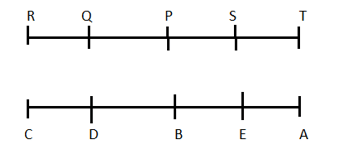# Reasoning Quiz for IBPS PO PRE 2019 | 02 September August 2019

## Reasoning Quiz for IBPS PO PRE

Reasoning Quiz to improve your  Reasoning  for SBI Po & SBI clerk exam Reasoning, IBPS PO Reasoning , IBPS Clerk Reasoning , IBPS RRB Reasoning, LIC AAO , LIC ADO,  and other competitive exams.

Directions (Q1-Q5): Study the following information to answer the given questions:

Ten people are sitting in two parallel rows containing five people each, in such a way that there is an equal distance between adjacent persons. In row 1, P, Q, R, S and T are seated and all of them are facing south. In row 2, A, B, C, D and E are seated and all of them are facing north. Therefore, in the given seating arrangement, each member seated in a row faces another member of the other row.

D sits third to the left of A. P faces immediate neighbor of D. R sits second to the right of P. Only one person sits between Q and S. B and E are immediate neighbors. E does not face P and Q.

Q1.How many persons are seated between Q and T?

(a) None

(b) One

(c) Two

(d) Three

(e) Cannot be determined

Q (1 – 5):Q1. Ans.(c)

P and S

Q2.Four of the following five are alike in a certain way and thus form a group. Which is the one that does not belong to that group?

(a)R

(b) S

(c)C

(d) T

(e)A

Q (1 – 5):Q2. Ans.(b)

All other are sitting at the ends.

Q3. Who among the following are sitting exactly in the middle of the rows?

(a)P,E

(b) S, D

(c) S, A

(d)A, R

(e) P, B

Q (1 – 5):Q3. Ans.(e)

Q4. Which of the following is true regarding B?

(a) A and C are immediate neighbors of B.

(b) B sits at one of the extreme ends of the line.

(c) Q faces B.

(d) T is an immediate neighbor of the person facing B.

(e) D sits on the immediate left of B.

Q (1 – 5):Q4. Ans.(e)

Q5. Four of the following five are alike in a certain way and thus form a group. Which is the one that does not belong to that group?

(a) T— E

(b) Q — C

(c) S — B

(d)R—A

(e) P — D

Q (1 – 5):Q5. Ans.(d)

In all other, the second is the neighbor of the one facing the first.

Directions (Q6 – Q10): Read the following information carefully to answer the following questions.

Q6. Statement: A≤B=C>D≥E

Conclusion: I. B> E      II. A≤D

(a)If only conclusion I follows.

(b)If only conclusion II follows.

(c)If either conclusion I or conclusion II follows.

(d)If neither conclusion I nor conclusion II follows.

(e)If both conclusions I and II follow.

Q6. Ans.(a)

1. B>E(True)
2. A≤D(False)

Q7. Statement: A≤B=C>D≥E

Conclusion: I. A≤C       II.E<C

(a)If only conclusion I follows.

(b)If only conclusion II follows.

(c)If either conclusion I or conclusion II follows.

(d)If neither conclusion I nor conclusion II follows.

(e)If both conclusions I and II follow.

Q7. Ans.(e)

1. A≤C (True)

II.E<C(True)

Q8. Statement:  A≥B=C≥D=E≤F

Conclusion: I. A≥D     II.B=E

(a)If only conclusion I follows.

(b)If only conclusion II follows.

(c)If either conclusion I or conclusion II follows.

(d)If neither conclusion I nor conclusion II follows.

(e)If both conclusions I and II follow.

Q8. Ans.(a)

1. A≥D(True)

II.B=E(False)

Q9. Statement: A≥B=C≥D=E≤F

Conclusion: I. A<F   II.C≥E

(a)If only conclusion I follows.

(b)If only conclusion II follows.

(c)If either conclusion I or conclusion II follows.

(d)If neither conclusion I nor conclusion II follows.

(e)If both conclusions I and II follow.

Q9. Ans.(b)

1. A<F(False)

II.C≥E(True)

Q10. Statement: A<B≤C=D , M≥N>O<B

Conclusion: I. C<M    II. B≤D

(a)If only conclusion I follows.

(b)If only conclusion II follows.

(c)If either conclusion I or conclusion II follows.

(d)If neither conclusion I nor conclusion II follows.

(e)If both conclusions I and II follow.

Q10. Ans.(b)

1. C<M(False)
2. B≤D(True)

### You Can Read This Also:

Best E-books for LIC AAO 2019 : Get PDF here

ambitiousbaba.com need your support to Grow

I challenge you will get Best Content in Our PDFs with Detail solutions and Latest Pattern

Memory Based Puzzle E-book | 2016-19 Exams Covered

Get PDF here

Caselet Data Interpretation 200 Questions

Get PDF here
Puzzle & Seating Arrangement E-Book for BANK PO MAINS (Vol-1)

Get PDF here

### ARITHMETIC DATA INTERPRETATION 2019 E-book

Get PDF here
The Banking Awareness 500 MCQs E-book| Bilingual (Hindi + English)

Get PDF here

High Level DATA INTERPRETATION Practice E-BOOK

Get PDF here

### How to Access on App:-

1. Go to Playstore search Ambitious Baba or Click here to Install App
2. After Install Login with Google Account or Facebook Account
3